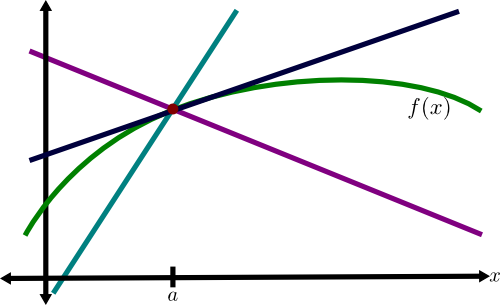# Math Insight

### Image: Insufficient condition for tangent lineThe condition that $\lim_{x\to a} |f(x)-L(x)|=0$ is clearly insufficient to specify a linear approximation $L(x)$ to the function $f(x)$ (shown in green) around the point $x=a$. Many linear functions (other colors) with many different slopes satisfy this condition, but only one (shown in dark blue) is tangent to the graph.

Image file: insufficient_condition_tangent_line.png

Source image file: insufficient_condition_tangent_line.svg
Source image type: Inkscape SVG